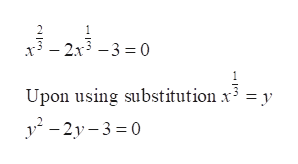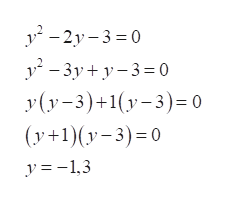x2/3-2x1/3-3=0solve by making an appropriate substitution

Question

x2/3-2x1/3-3=0

solve by making an appropriate substitution

Step 1

We have been given an equation with fractional exponents that we need to solve.

Step 2

In order to solve this equation, we can use the substitution x^1/3 = y. Upon doing so, we will get our equation as shown below:help_outlineImage Transcriptionclose- 2x3 -3 0 Upon using substitution x3 = y 2y30 fullscreen
Step 3

Upon solving this equation ...help_outlineImage Transcriptionclose-2y-3 0 -3y+ y-3 = 0 y(y3)1y0 (y+1)y3)0 = 0 y=-1,3 fullscreen

Want to see the full answer?

See Solution

Want to see this answer and more?

Our solutions are written by experts, many with advanced degrees, and available 24/7

See Solution
Tagged in

Equations and In-equations